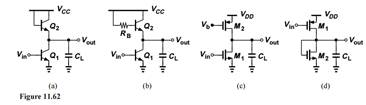### Create an Account

Home / Questions / Determine the 3-dB bandwidth of the circuits shown in Fig 11 62 Assume VA ∞ but λ 0. Negl...

# Determine the 3-dB bandwidth of the circuits shown in Fig 11 62 Assume VA ∞ but λ 0. Neglect other capacitances

Determine the 3-dB bandwidth of the circuits shown in Fig. 11.62. Assume VA = ∞ but λ > 0. Neglect other capacitances.Apr 24 2020 View more View LessSubscribe To Get Solution